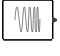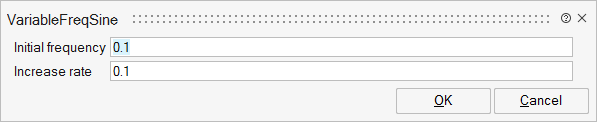# VariableFreqSine

This block is a variable frequency sinewave generator.SignalGenerators

## Description

This block generates a variable frequency sinewave. Initial frequency and its variation across time (Increase rate) are specified as block parameters.

The frequency is changing linearly starting from Initial frequency and increases with the Increase rate.If we denote F the Initial frequency and R the Increase rate, the output of the block is computed as follows:Sin(2*PI*(F+R*time/2)*time)

## ParametersNameLabelDescriptionData TypeValid Values

FInit

Initial frequency

Initial Frequency of the sine wave. Can be a scalar (one signal) or a vector (multiple signals are generated).

Matrix

IncreaseRate

Increase rate

Variation rate (can be either positive or negative) of the sine wave. If scalar, applies to all signals. If a vector, applies to each signal vector - provided size matches the size of the Initial frequency vector.

Matrix

## Ports

NameTypeDescriptionIO TypeNumber

Port 1

explicit

output

1

NameValueDescription

always active

yes

Time Dependency = standard mode activated

direct-feedthrough

no

zero-crossing

no

mode

no

continuous-time state

no

discrete-time state

no